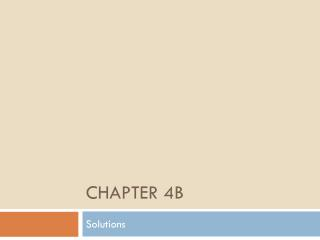DownloadDownload PresentationChapter 4B

# Chapter 4B

Télécharger la présentation## Chapter 4B

- - - - - - - - - - - - - - - - - - - - - - - - - - - E N D - - - - - - - - - - - - - - - - - - - - - - - - - - -
##### Presentation Transcript

1. Chapter 4B Solutions

2. Solutions Homogeneous mixture • Solvent – substance present in the largest amount • Solute – substance present in the smallest amount Aqueous solution – solutions with water as the solvent.

3. Types of Solutions

4. Solubility The maximum amount of a solute that can be dissolved in a given amount of solvent at a constant temperature. Factors that affect rate of Solubility (increase the rate of dissolving). • Pressure • Temperature • Polarity

5. Solubility Solutions can be classified into groups by how much solute is in solution. • Saturated – contains as much solute as the solvent can hold at a given temperature. • Unsaturated – less the maximum amount of solvent at a given temperature. • Supersaturated – contains more solute than the solvent can hold at a given temperature.

6. Solution by Mass Percent Solutions are described by mass percent

7. Solutions by Mass Percent A solution is prepared by mixing 2.50 grams of calcium chloride with 50.0 grams of water. Calculate the mass percent of calcium chloride in this solution.

8. Solutions by Mass Percent A 75.0 gram sample of a solution is known to contain 23.8 grams of glucose. Calculate the mass percent of glucose in this solution.

9. Solutions by Mass Percent Vinegar is a solution of acetic acid in water and typically contains 5.00% acetic acid by mass. Calculate the mass of acetic acid in a 250.0 gram sample of vinegar.

10. Solutions by Mass Percent Concentrated hydrochloric acid solution contains 37.2 % by mass HCl. What mass of HCl is contained in 35.5 grams of concentrated HCl.

11. Molarity Molarity – concentration of a solution

12. Molarity Calculate the molarity of a solution prepared by dissolving 15.6 gram of solid KBr in enough water to make 1.25 L of solution.

13. Molarity Calculate the molarity of a solution prepared by dissolving 2.80 grams of solid NaCl in enough water to make a 135 ml of solution

14. Molarity What mass of NaNO3 is required to make 2.50 Liter of a 0.150 M solution of NaNO3?

15. Molarity What mass of (NH4)2SO4 is required to make 1.25 liter of 0.250 M solution of (NH4)2SO4?

16. Molarity-Ion Concentration Give the concentrations of all ions in each of the following solutions • 1.20 M Na2SO4 • 0.750 M K2CrO4

17. Molarity-Ion Concentration How many moles of Na+ ions are present in 42.0 ml of a 0.350 M NaCl solution?

18. Molarity-Ion Concentration How many moles of Na+ ions are present in 42.0 ml of a 0.350 M of Na3PO4 solution?

19. Dilutions Process of adding more solvent to a solution. M1=molarity before dilution V1=volume before dilution M2=molarity after dilution V1=volume after dilution

20. Dilution What volume of 19 M NaOH must be used to prepare a 1.0 Liter of a 0.15 M NaOH solution?

21. Dilution What volume of water is needed to prepare 500.0 ml of a 0.250 M Ca(NO3)2 solution from a 5.00 M solution of Ca(NO3)2?

22. Titrations Combining a solution of know concentration with a solution of unknown concentration to determine the unknown. • Equivalence point – when the reactants are equal molar. • Indicator – dyes used in titrations to indicate equivalence point.

23. Titrations • What volume of 0.115 M HCO4 solution is needed to neutralize 50.00 ml of 0.0875 M NaOH? • 15.00 ml of acetic acid is titrated with 0.500 M NaOH. It takes 22.59 ml of NaOH. What is the molarity of the acetic acid? • How many grams of AgBr are formed when 35.5 ml of 0.184 M AgNO3 are mixed with an excess of aqueous hydrobromic acid?